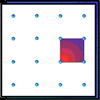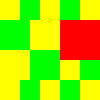You may also likeGeoboards

This practical challenge invites you to investigate the different squares you can make on a square geoboard or pegboard.Tiles on a Patio

How many ways can you find of tiling the square patio, using square tiles of different sizes?Pebbles

Place four pebbles on the sand in the form of a square. Keep adding as few pebbles as necessary to double the area. How many extra pebbles are added each time?

A Day with Grandpa

Age 7 to 11 Challenge Level:

It is easy to get confused by these sorts of calculations. Fortunately Felipe from Quarry Bay School and Toby, Liam, Abi and Livvy from Shute Community Primary School didn't get confused, and all came up with the correct solutions.

The first rug measures $2$ yards $1$ foot by $1$ yards $1$ foot.

$2$ yards $1$ foot = $(2\times 3) + 1 = 7$ feet and $1$ yard $1$ foot = $(1\times 3) + 1 = 4$ feet. Therefore the area of the rug is $7\times 4 = 28$ square feet.

Many of you correctly realised that if $1$ yard is $3$ feet then $1$ square yard is $3\times 3 = 9$ square feet. $28 = 3\times 9 + 1 = 3$ square yards $1$ square foot. So Grandad was correct.

Grandad was also correct the second time:

$2$ feet $6$ inches $\times 1$ foot $3$ inches

$= (2\times 12) + 6$ inches $\times (1\times 12) + 3$ inches

$= 30\times 15$ square inches

$= 450$ square inches

$= (3\times 144) + 18$ square inches

$= (3\times 12^2) + 18$ square inches

$= 3$ square feet $18$ square inches.

We work out the last one like this:

$2$ yards $1$ foot $5$ inches $\times 1$ yard $2$ feet $7$ inches

$= (2\times 3) + 1$ feet $5$ inches $\times (1\times 3) + 2$ feet $7$ inches

$= 7$ feet $5$ inches $\times 5$ feet $7$ inches

$= (7\times 12) + 5$ inches $\times (5\times 12) + 7$ inches

$= 89$ inches $\times 67$ inches

$= 89\times 67 = 5963$ square inches

$= (41\times 144) + 59$ square inches

$= 41$ square feet $59$ square inches

$= (4\times 9) + 5$ square feet $59$ square inches

$= 4$ square yards $5$ square feet $59$ square inches